# Search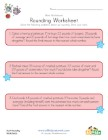## Patriotic Rounding Worksheet

Practice rounding with a fun patriotic theme.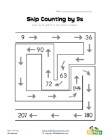## Skip Counting by Nine Worksheet

Learn to skip count by nines with these printable ...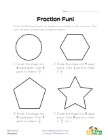## Fraction Fun Worksheet 2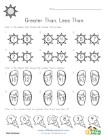## Summer Greater, Less Than Coloring Worksheet

Follow the instructions and color the suns, sandal...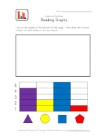## Graphing Worksheet

Use the bar graph to determine how many of each sh...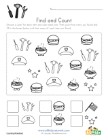## Labor Day Find and Count Worksheet

Find and count the flags, stars, tools and more.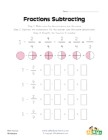## Simple Fraction Subtraction Worksheet 3

A slightly more involved, but still simple, fracti...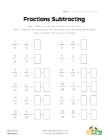## Simple Fraction Subtraction Worksheet 4

12 simple subtracting fractions problems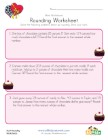## Valentine's Day Rounding Worksheet

Practice rounding with a fun Valentine's Day theme...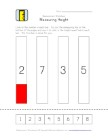## Measurement Worksheet

Cut out and use the measurement bar to help color ...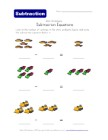## Subtraction Equations Worksheet - Vehicles Theme

Count the vehicles and write the basic subtraction...